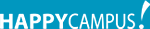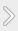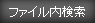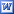#### タグ

レポート理工学運動量エネルギー仕事力学物理

### 資料紹介

Objectives:

Use a Motion Detector and a Force Sensor to measure the position and force on a hanging mass, a spring, and a dynamics cart. Then determine the work done on an object using a force vs. position graph. Work can determined graphically as the area under the plot of force vs. position.

Analysis Q.1
Yes, the work done on the mass corresponds to the change in gravitational potential energy and it should be. Potential energy exists when an object (with mass) has a position within a force field so in this experiment, mass is in the position of objects in the earth's gravitational field. Kinetic Energy exists when an object (with mass) is in motion with some velocity. In Part I, lifting the mass didn’t change its kinetic energy so use equation and it can say that the work done on the mass corresponds to the change in gravitational potential energy.

### 資料の原本内容( この資料を購入すると、テキストデータがみえます。 )

*Work and Energy
Objectives:
Use a Motion Detector and a Force Sensor to measure the position and force on a hanging mass, a spring, and a dynamics cart. Then determine the work done on an object using a force vs. position graph. Work can determined graphically as the area under the plot of force vs. position.
・Work = Force × Displacement
・Work = △Potential Energy ＋ △Kinetic Energy
・△Potential Energy = Mass × Gravity (approximately 10ms^-2) × △Height
・△Kinetic Energy = 1/2 × Mass × (Velocity)^2..

## featherの資料(176)

•Relax
•Report image
•Simple and Stylish
•Stylish green
•Vision

### コメント追加

コメントを書込むには会員登録するか、すでに会員の方はログインしてください。